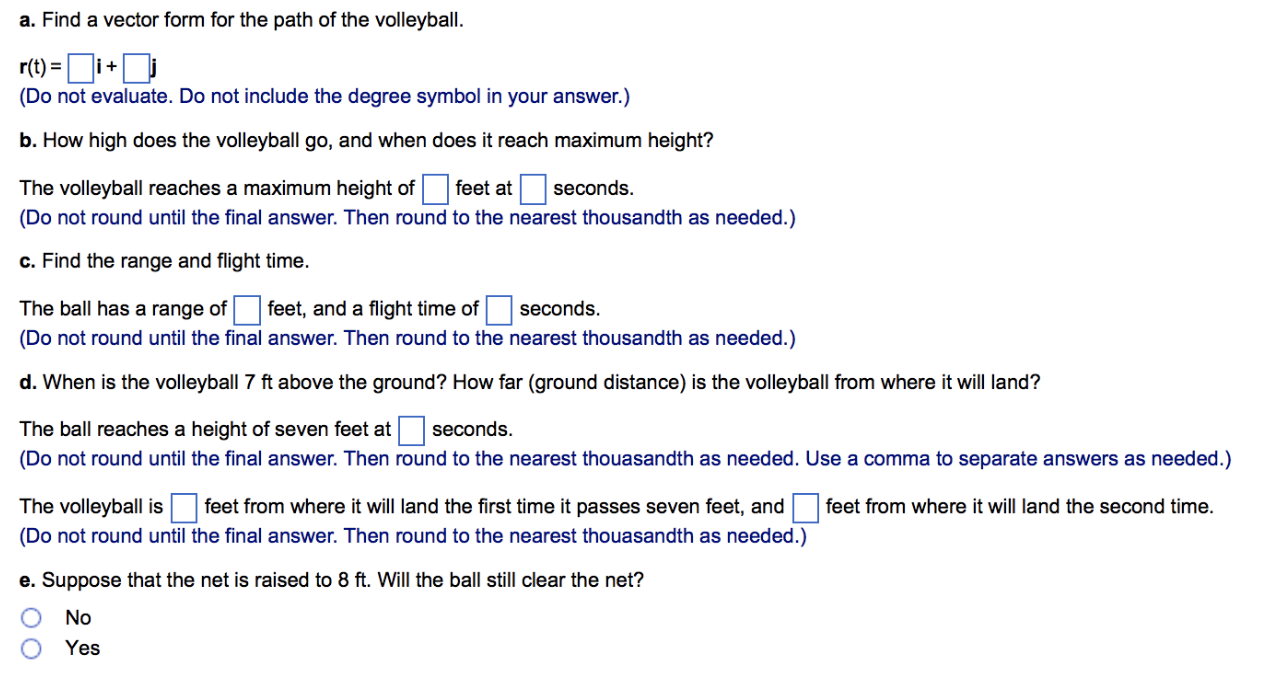Home / Answered Questions / Other / a-volleyball-is-hit-when-it-is-4-ft-above-the-ground-and-12-ft-from-a-6-ft-high-net-it-leaves-the-po-aw401

# (Solved): A Volleyball Is Hit When It Is 4 Ft Above The Ground And 12 Ft From A 6-ft-high Net. It Leaves The P...A volleyball is hit when it is 4 ft above the ground and 12 ft from a 6-ft-high net. It leaves the point of impact with an initial velocity of 47 ft/sec at an angle of 31Â° and slips by the opposing team untouched. The acceleration due to gravity is g=32 ft/sec2. Answer the following questions. a. Find a vector form for the path of the volleyball. (Do not evaluate. Do not include the degree symbol in your answer.) b. How high does the volleyball go, and when does it reach maximum height? The volleyball reaches a maximum height of feet at seconds. (Do not round until the final answer. Then round to the nearest thousandth as needed.) c. Find the range and flight time. The ball has a range of feet, and a flight time of seconds. (Do not round until the final answer. Then round to the nearest thousandth as needed.) d. When is the volleyball 7 ft above the ground? How far (ground distance) is the volleyball from where it will land? The ball reaches a height of seven feet at seconds. (Do not round until the final answer. Then round to the nearest thouasandth as needed. Use a comma to separate answers as needed.) feet from where it will land the second time. The volleyball is feet from where it will land the first time it passes seven feet, and (Do not round until the final answer. Then round to the nearest thouasandth as needed.) e. Suppose that the net is raised to 8 ft. Will the ball still clear the net? ÎŸ ÎÎ¿ O Yes

We have an Answer from Expert